Collinearity Of two vectors

Chapter 10 Class 12 Vector Algebra
Concept wise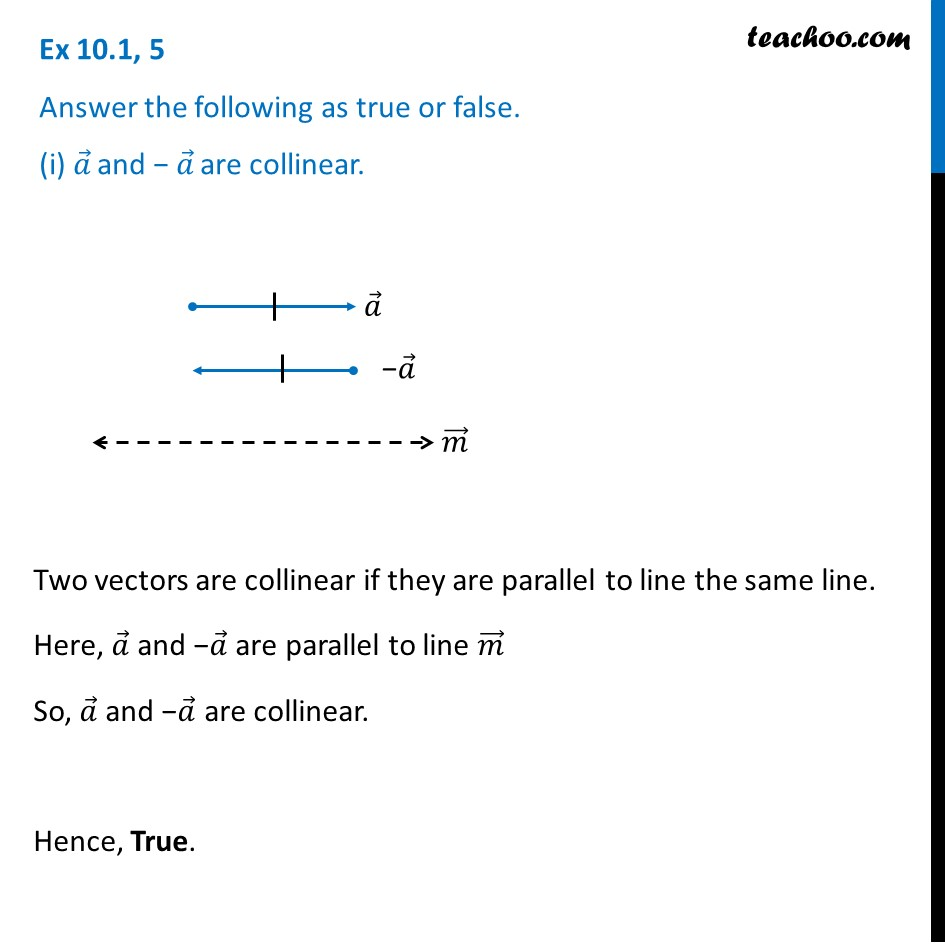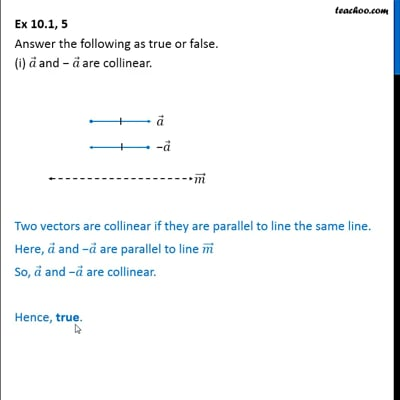This video is only available for Teachoo black users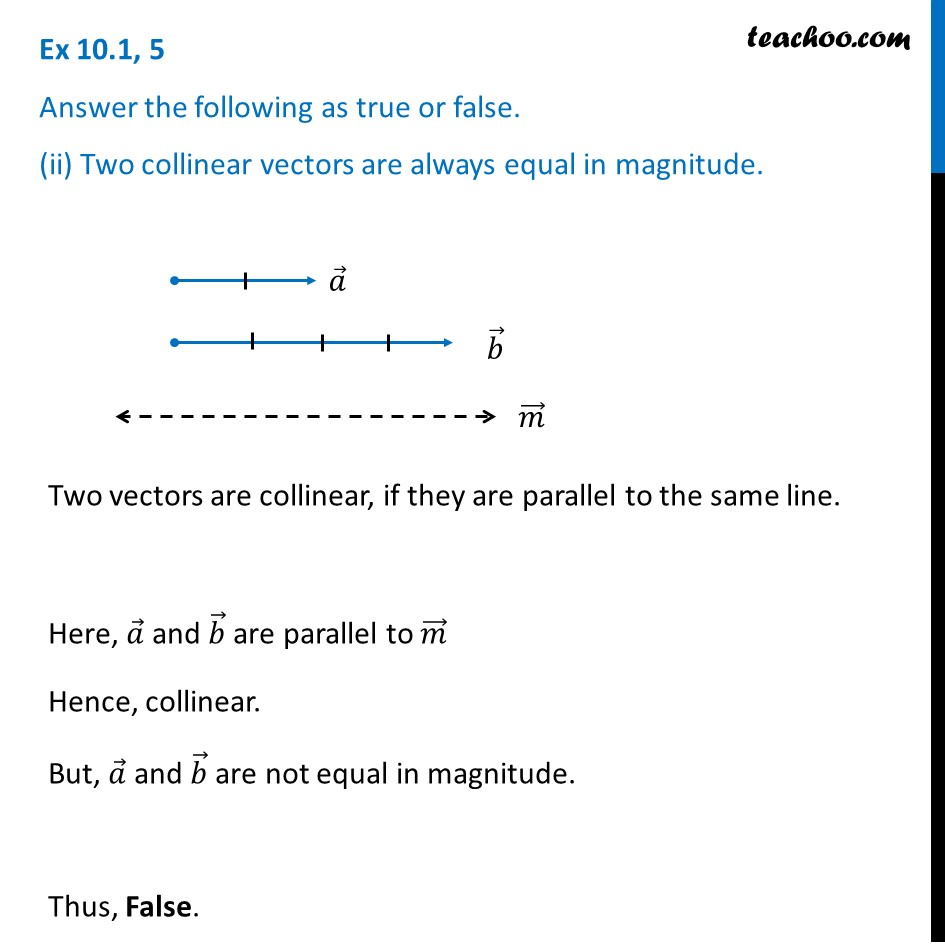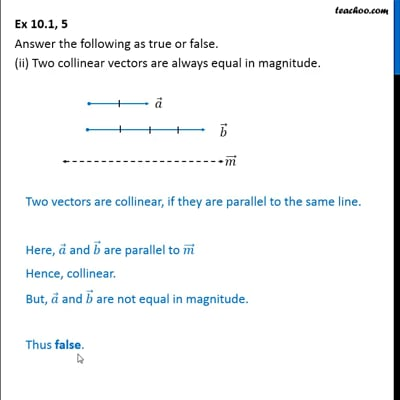This video is only available for Teachoo black users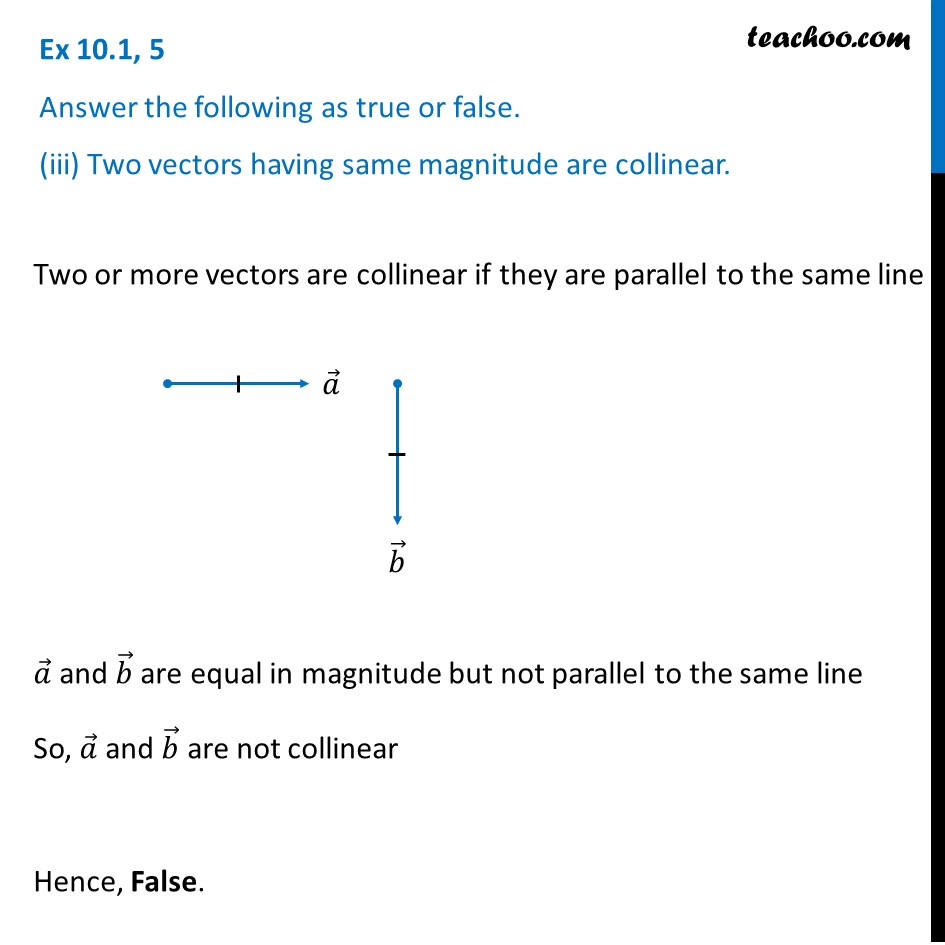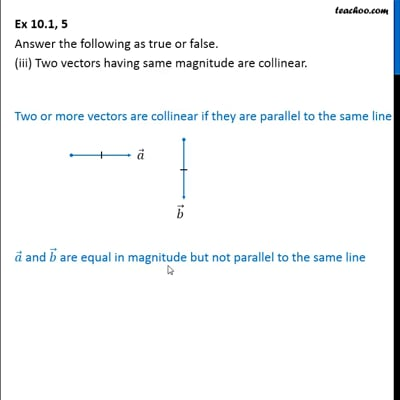This video is only available for Teachoo black users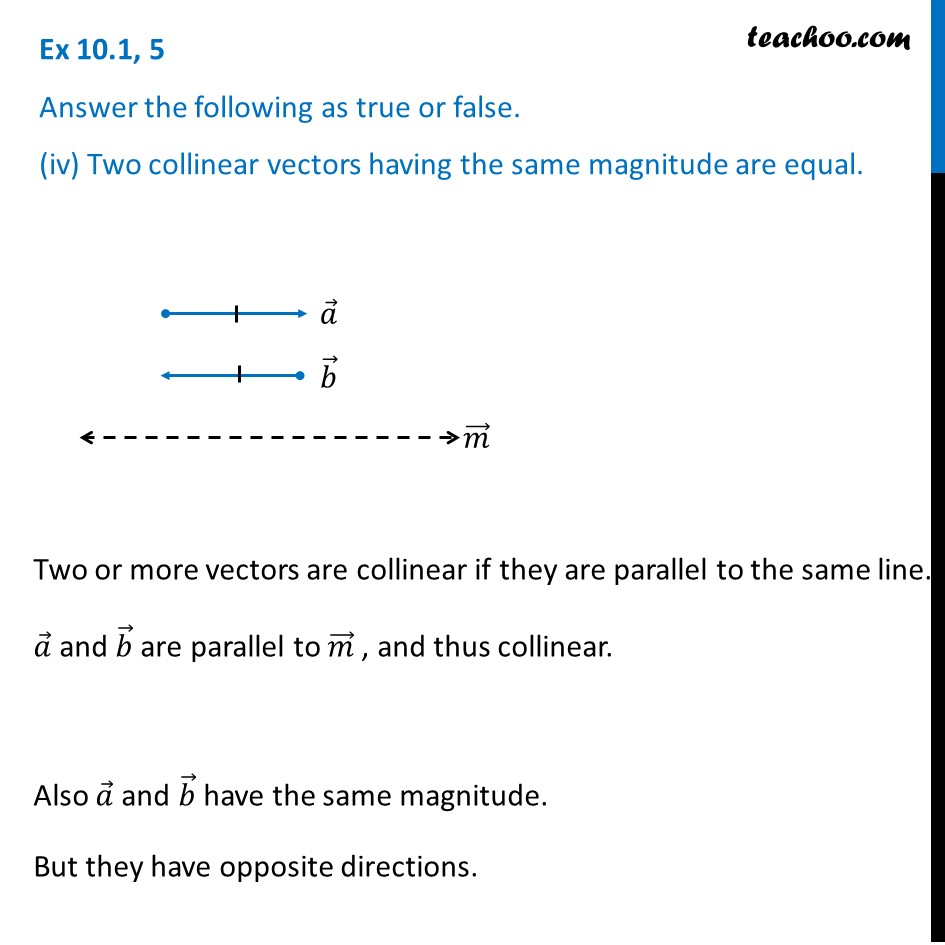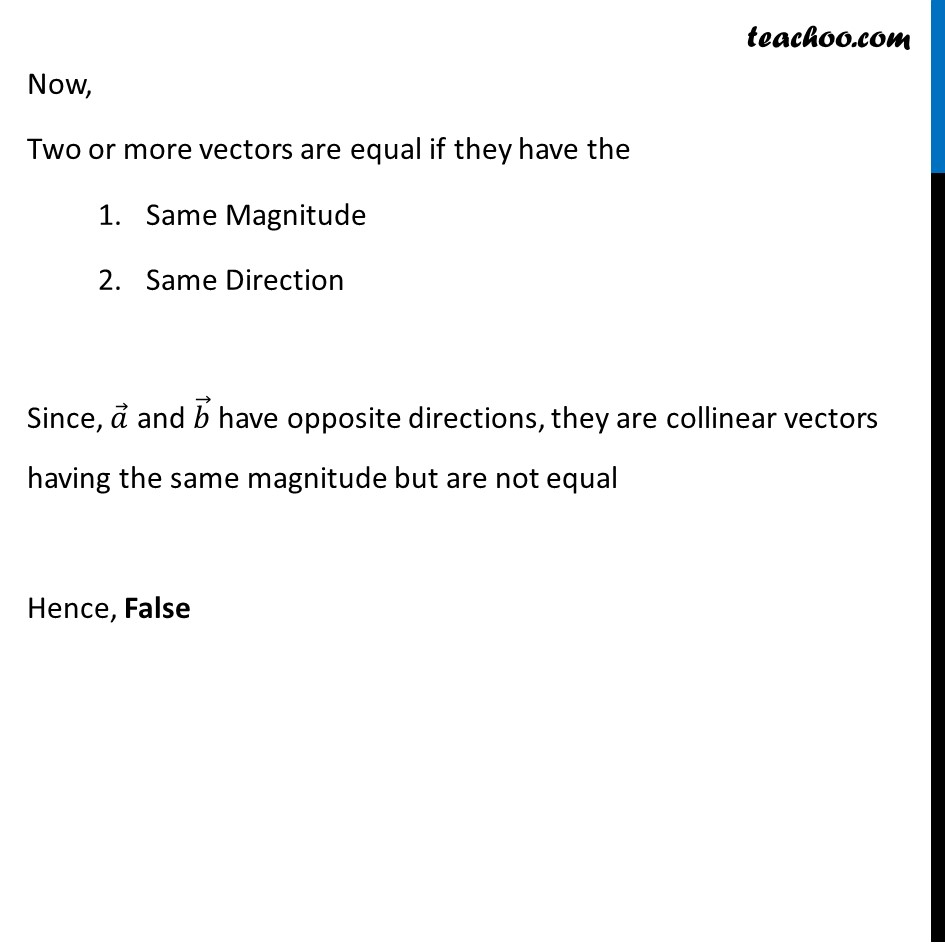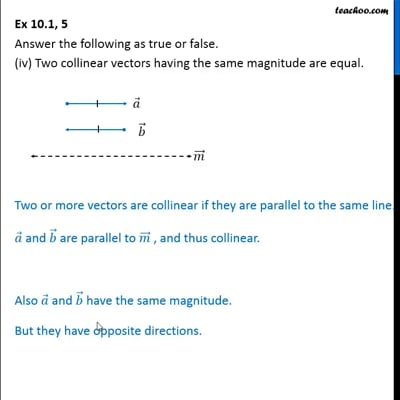This video is only available for Teachoo black users

Introducing your new favourite teacher - Teachoo Black, at only ₹83 per month

### Transcript

Ex 10.1, 5 Answer the following as true or false. (i) 𝑎 ⃗ and − 𝑎 ⃗ are collinear.Two vectors are collinear if they are parallel to line the same line. Here, 𝑎 ⃗ and −𝑎 ⃗ are parallel to line 𝑚 ⃗ So, 𝑎 ⃗ and −𝑎 ⃗ are collinear. Hence, True. Ex 10.1, 5 Answer the following as true or false. (ii) Two collinear vectors are always equal in magnitude.Two vectors are collinear, if they are parallel to the same line. Here, 𝑎 ⃗ and 𝑏 ⃗ are parallel to 𝑚 ⃗ Hence, collinear. But, 𝑎 ⃗ and 𝑏 ⃗ are not equal in magnitude. Thus, False. Ex 10.1, 5 Answer the following as true or false. (iii) Two vectors having same magnitude are collinear.Two or more vectors are collinear if they are parallel to the same line 𝑎 ⃗ and 𝑏 ⃗ are equal in magnitude but not parallel to the same line So, 𝑎 ⃗ and 𝑏 ⃗ are not collinear Hence, False. Ex 10.1, 5 Answer the following as true or false. (iv) Two collinear vectors having the same magnitude are equal.Two or more vectors are collinear if they are parallel to the same line. 𝑎 ⃗ and 𝑏 ⃗ are parallel to 𝑚 ⃗ , and thus collinear. Also 𝑎 ⃗ and 𝑏 ⃗ have the same magnitude. But they have opposite directions. Now, Two or more vectors are equal if they have the Same Magnitude Same Direction Since, 𝑎 ⃗ and 𝑏 ⃗ have opposite directions, they are collinear vectors having the same magnitude but are not equal Hence, False